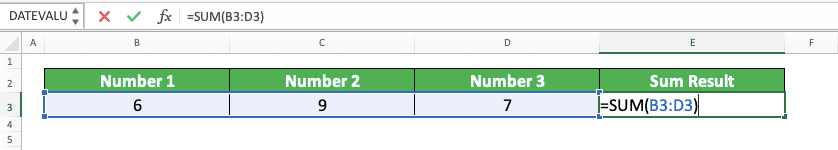The Complete Tutorial List of Excel Functions - Compute Expert

# The Complete Tutorial List of Excel Functions

Home >> Excel Tutorials from Compute Expert >> The Complete Tutorial List of Excel Functions

Want to master the usage of excel functions? Then, you have come to the right place!

Here, you can find a list containing the links to the Compute Expert’s in-depth tutorials for each function in excel. In each of the links, we add a brief explanation about the function’s usefulness. Thus, you will know what you can expect to do if you master the function usage in excel.

Before we go to the complete list, we will discuss a bit about the details of an excel function. From the discussion, you should understand its definition, benefits, and how to add a function formula to your cell generally.

## What is an Excel Function?

An excel function is a feature excel provides to help us in processing our data into our desired outputs. Excel has many function variations and each of them has a unique usefulness that can perform a specific task for us.

## The Benefits of Using Excel Functions

There are many benefits we will get if we can use functions optimally in excel. Each function has its own unique benefit related to data processing. However, generally, here are the main benefits of functions in excel.
• Get our desired outputs fast and accurately from the data that we have
• Create the materials we need for further data processing
• Automate our data processing if the data we work with in excel is dynamic

Those benefits are crucial if we want to process our data productively in excel. Therefore, try to master as many functions as possible if you often do your work in excel!

## How to Add a Function Formula in Excel

When you want to write a function formula in excel, you have to type an equal symbol ( = ) before you write it. The symbol will inform excel that you want to write some kind of formula in your cell.

After the equal symbol, you should write the name of the function you want to use. Next, give the inputs you need to use the function inside bracket signs. Each function needs different kinds of inputs to produce the result that you want from it.

For example, here is a SUM function that we write to sum numbers in a cell range. Notice that the formula writing follows the pattern we discussed earlier.Another way to add a function in excel is by using the Insert Functions menu in the Formulas tab. You can see the menu location in the screenshot below.You can click the Insert Functions button and choose the function you want to use on its dialog box. Alternatively, you can also click the dropdown of the function type you want to use in the Formulas tab. Then, just choose the function from the list that shows up.

If you insert your function using the Insert Functions menu, there will be formula writing helpers from excel you can use. Insert the inputs that the function needs to the text boxes excel gives and excel will write the formula for you.

## The Complete Tutorial List of Excel Functions

Even with the help of the Insert Functions menu, though, many people still find it hard to use excel functions correctly. Because of that, Compute Expert provides tutorials on how to use excel functions on its website!

You can see the full list of the tutorials we have already written below. We list the tutorials alphabetically so you can find the function you want to learn easily.

Each of our tutorials contains an in-depth discussion on a specific function that becomes the tutorial topic. We also provide simple exercises so you can practice what you have learned from the tutorial. Hopefully, the content is enough for you to understand the function usage you wish to learn completely!

Get updated excel info from Compute Expert by registering your email. It's free!